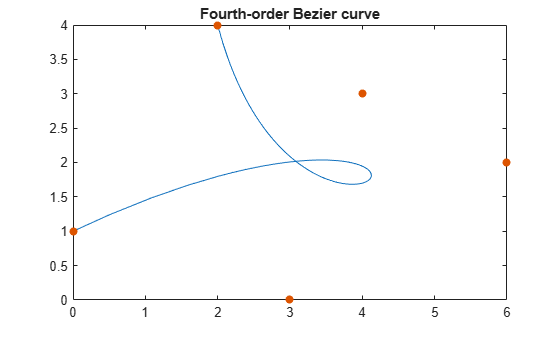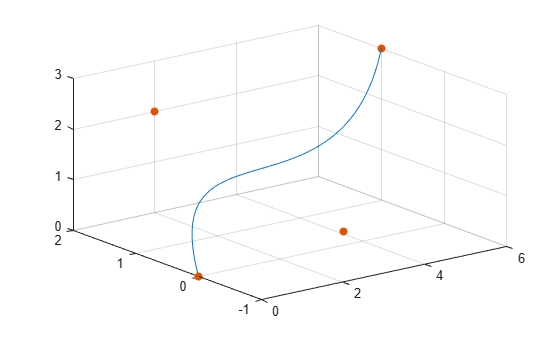Main Content

# bernsteinMatrix

Bernstein matrix

## Syntax

``B = bernsteinMatrix(n,t)``

## Description

example

````B = bernsteinMatrix(n,t)`, where `t` is a vector, returns the `length(t)`-by-`(n+1)` Bernstein matrix `B`, such that `B(i,k+1)= nchoosek(n,k)*t(i)^k*(1-t(i))^(n-k)`. Here, the index `i` runs from 1 to `length(t)`, and the index `k` runs from `0` to `n`.The Bernstein matrix is also called the Bezier matrix.Use Bernstein matrices to construct Bezier curves:bezierCurve = bernsteinMatrix(n, t)*PHere, the `n+1` rows of the matrix `P` specify the control points of the Bezier curve. For example, to construct the second-order 3-D Bezier curve, specify the control points as:P = [p0x, p0y, p0z; p1x, p1y, p1z; p2x, p2y, p2z]```

## Examples

collapse all

Plot the fourth-order Bezier curve specified by the control points `p0 = [0 1]`, `p1 = [4 3]`, `p2 = [6 2]`, `p3 = [3 0]`, `p4 = [2 4]`. Create a matrix with each row representing a control point.

`P = [0 1; 4 3; 6 2; 3 0; 2 4];`

Compute the fourth-order Bernstein matrix `B`.

```syms t B = bernsteinMatrix(4,t);```

Construct the Bezier curve.

`bezierCurve = simplify(B*P);`

Plot the curve adding the control points to the plot.

```fplot(bezierCurve(1), bezierCurve(2), [0, 1]) hold on scatter(P(:,1), P(:,2),'filled') title('Fourth-order Bezier curve') hold off```Construct the third-order Bezier curve specified by the 4-by-3 matrix `P` of control points. Each control point corresponds to a row of the matrix `P`.

`P = [0 0 0; 2 2 2; 2 -1 1; 6 1 3];`

Compute the third-order Bernstein matrix.

```syms t B = bernsteinMatrix(3,t);```

Construct the Bezier curve.

`bezierCurve = simplify(B*P);`

Plot the curve adding the control points to the plot.

```fplot3(bezierCurve(1), bezierCurve(2), bezierCurve(3), [0, 1]) hold on scatter3(P(:,1), P(:,2), P(:,3),'filled') hold off```Construct the third-order Bezier curve with the evaluation point specified by the following `1`-by-`101` vector `t`.

`t = 0:1/100:1;`

Compute the third-order `101`-by-`4` Bernstein matrix and specify the control points.

```B = bernsteinMatrix(3,t); P = [0 0 0; 2 2 2; 2 -1 1; 6 1 3];```

Construct and plot the Bezier curve. Add grid lines and control points to the plot.

```bezierCurve = B*P; plot3(bezierCurve(:,1), bezierCurve(:,2), bezierCurve(:,3)) hold on grid scatter3(P(:,1), P(:,2), P(:,3),'filled') hold off```## Input Arguments

collapse all

Approximation order, specified as a nonnegative integer.

Evaluation point, specified as a number, symbolic number, variable, expression, or vector.

## Output Arguments

collapse all

Bernstein matrix, returned as a `length(t)`-by-`n+1` matrix.

## See Also

Introduced in R2013b

## Support

#### Mathematical Modeling with Symbolic Math Toolbox

Get examples and videos